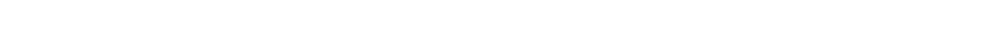# 1.6.7 Bond Enthalpies

### Bond Enthalpies

• The amount of energy required to break one mole of a specific covalent bond in the gas phase is called the bond dissociation energy
• Bond dissociation energy (E) is usually just simplified to bond energy or bond enthalpy
• In symbols, the type of bond broken is written in brackets after E
• Eg. E (H-H) is the bond energy of a mole of single bonds between two hydrogen atoms

#### Average bond energy

• Bond energies are affected by other atoms in the molecule (the environment)
• Therefore, an average of a number of the same type of bond but in different environments is calculated
• This bond energy is known as the average bond energy
• Since bond energies cannot be determined directly, enthalpy cycles are used to calculate the average bond energy

#### Calculating enthalpy change from bond energies

• Bond energies are used to find the ΔHr of a reaction when this cannot be done experimentally
• The formula is:The formula for calculating the standard enthalpy change of reaction using bond energies

#### Worked Example

Calculating the enthalpy change in the Haber process

Calculate the change in enthalpy of reaction for the Haber process, producing ammonia from hydrogen and nitrogen:

N2 (g) + 3H2 (g) ⇌ 2NH3 (g)

The relevant bond energies are given in the table below:Step 1: Use the equation to work out the bonds broken and formed and set out the calculation as a balance sheet as shown below:

N2 (g) + 3H2 (g) ⇌ 2NH3 (g)Note! Values for bonds broken are positive (endothermic) and values for bonds formed are negative (exothermic)

Step 2: Calculate the standard enthalpy of reaction

ΔHr = enthalpy change for bonds broken + enthalpy change for bonds formed

= (+2253 kJ mol-1) + (-2346 kJ mol-1)

= -93 kJ mol-1

#### Worked Example

Calculating the enthalpy of combustion using bond enthalpies

The complete combustion of ethyne, C2H2, is shown in the equation below:Using the average bond enthalpies given in the table, what is the enthalpy of combustion of ethyne?Step 1: The enthalpy of combustion is the enthalpy change when one mole of a substance reacts in excess oxygen to produce water and carbon dioxide

The chemical reaction should be therefore simplified such that only one mole of ethyne reacts in excess oxygen:

H-C≡C-H + 2 ½ O=O → H-O-H + 2O=C=O

Step 2: Set out the calculation as a balance sheet as shown below:ΔHr = enthalpy change for bonds broken + enthalpy change for bonds formed

= (+2912 kJ mol-1) + (- 4142 kJ mol-1)

= -1230 kJ mol-1

CloseClose